Case Based Questions (MCQ)

Chapter 10 Class 12 Vector Algebra
Serial order wise

## Solar Panels have to be installed carefully so that the tilt of the roof, and the direction to the sun, produce the largest possible electrical power in the solar panels. A surveyor uses his instrument to determine the coordinates of the four corners of a roof where solar panels are to be mounted. In the picture , suppose the points are labelled counter clockwise from the roof corner nearest to the camera in units of meters P 1 (6, 8, 4) , P 2 (21, 8, 4), P 3 (21, 16, 10) and P 4 (6, 16, 10)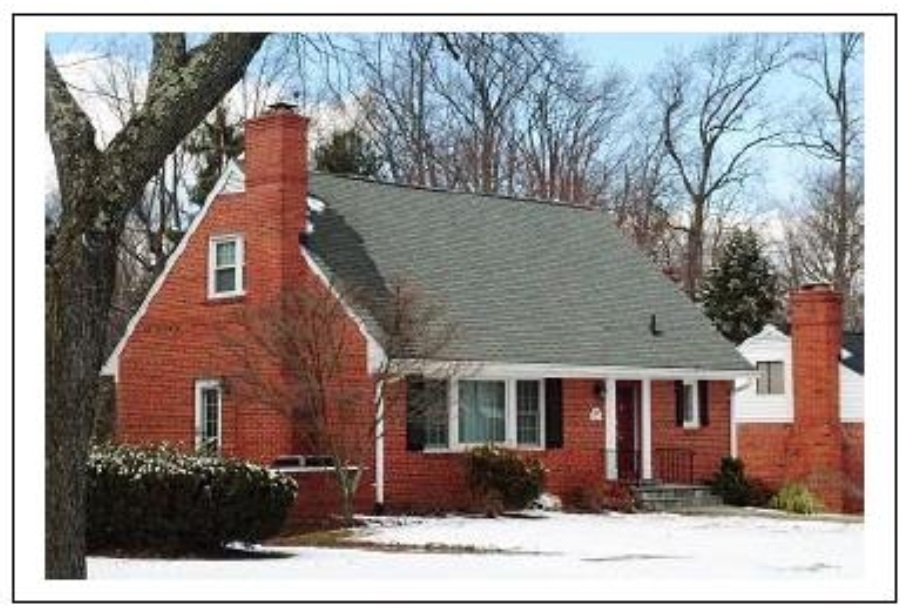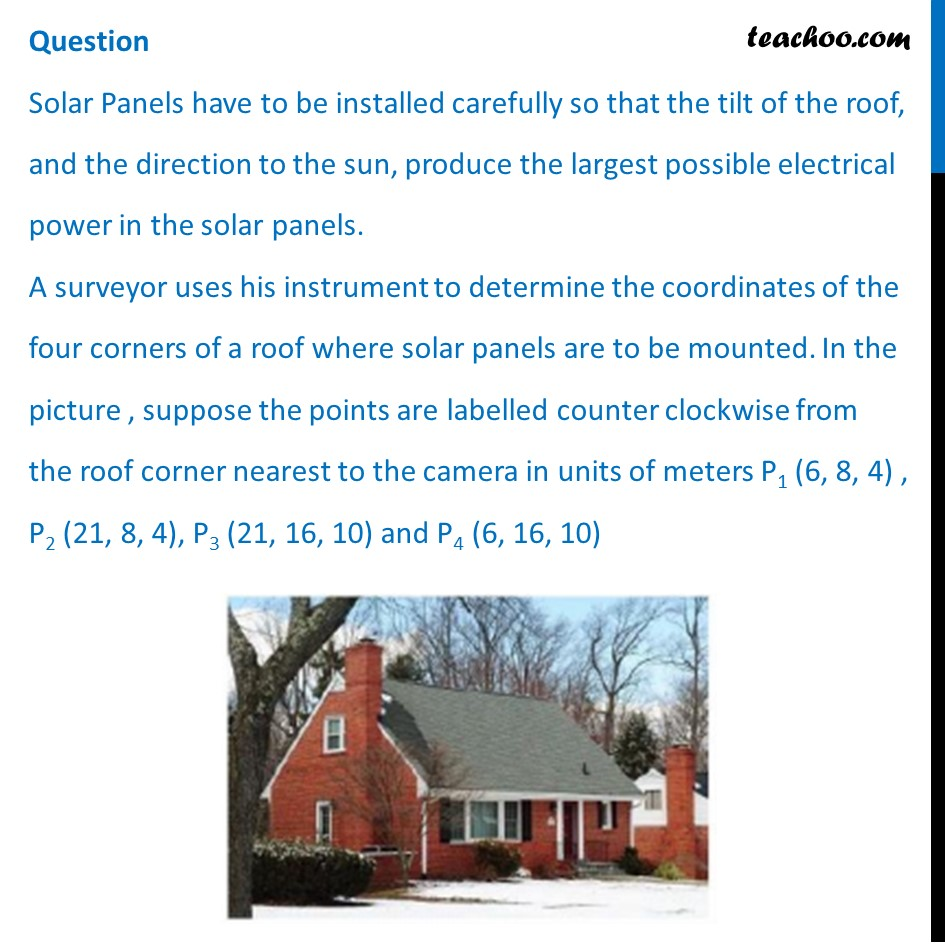## What are the components to the two edge vectors defined by A ⃗ = PV of P 2 – PV of P1 and B ⃗  = PV of P 4 – PV of P 1 ? (where PV stands for position vector)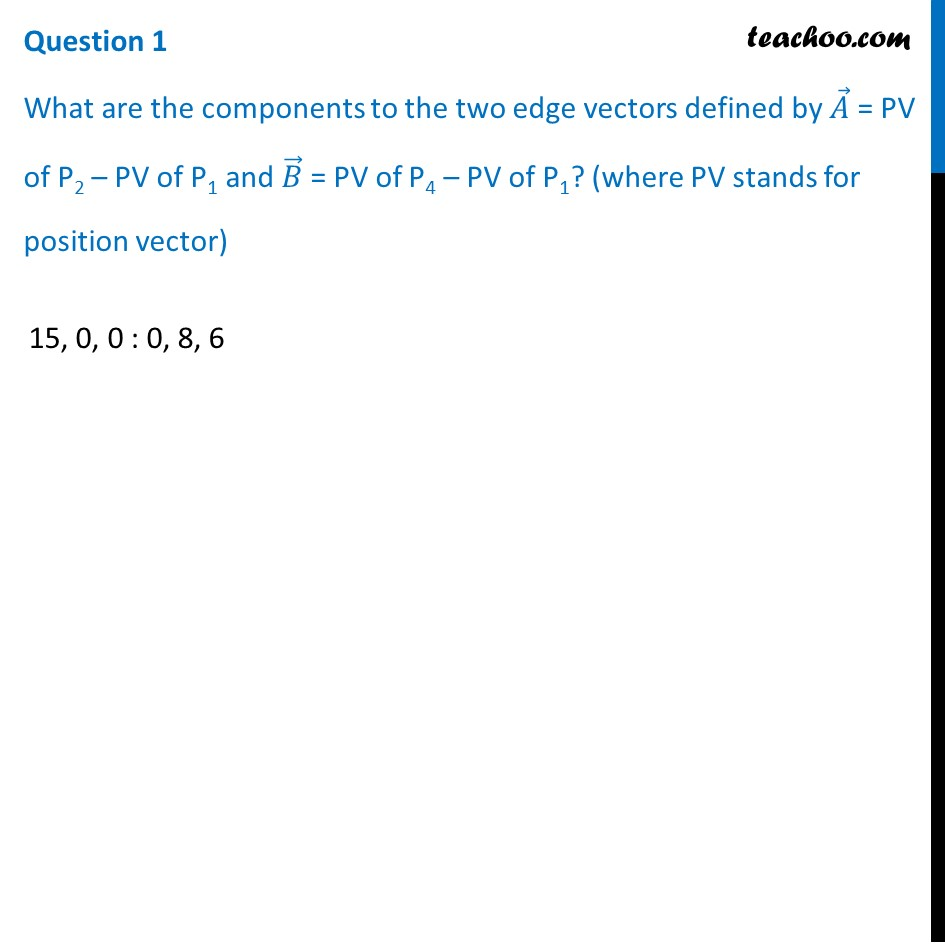## Write the vector in standard notation with i ̂, j ̂  and k ̂ (where i ̂, j ̂  and k ̂ are the unit vectors along the three axes).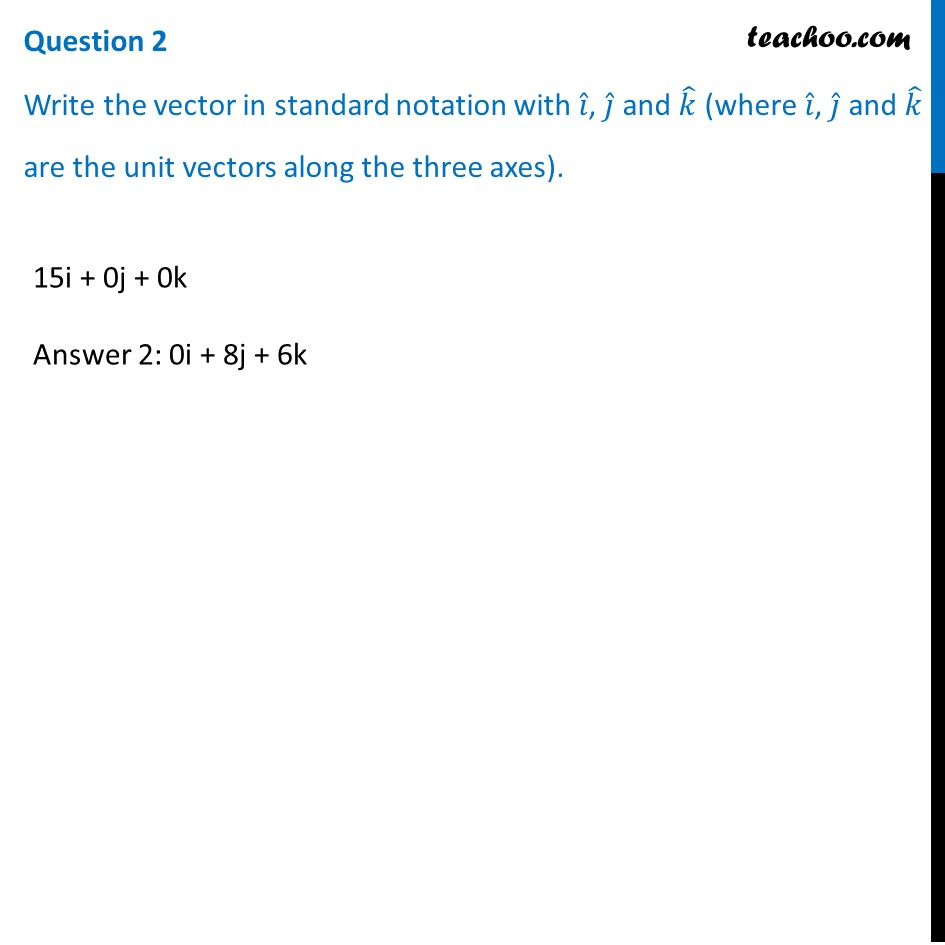## What are the magnitudes of the vectors A ⃗ and B ⃗ and in what units?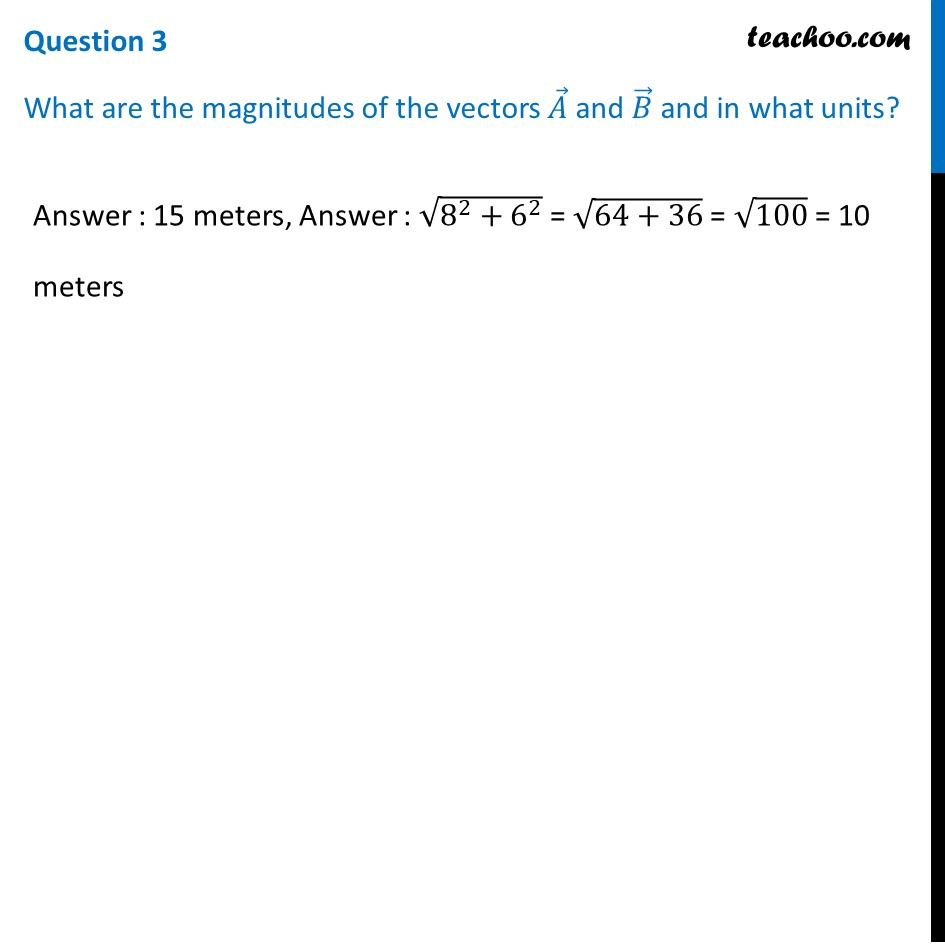## What are the components to the vector N ⃗ , perpendicular to A ⃗ and B ⃗ and the surface of the roof?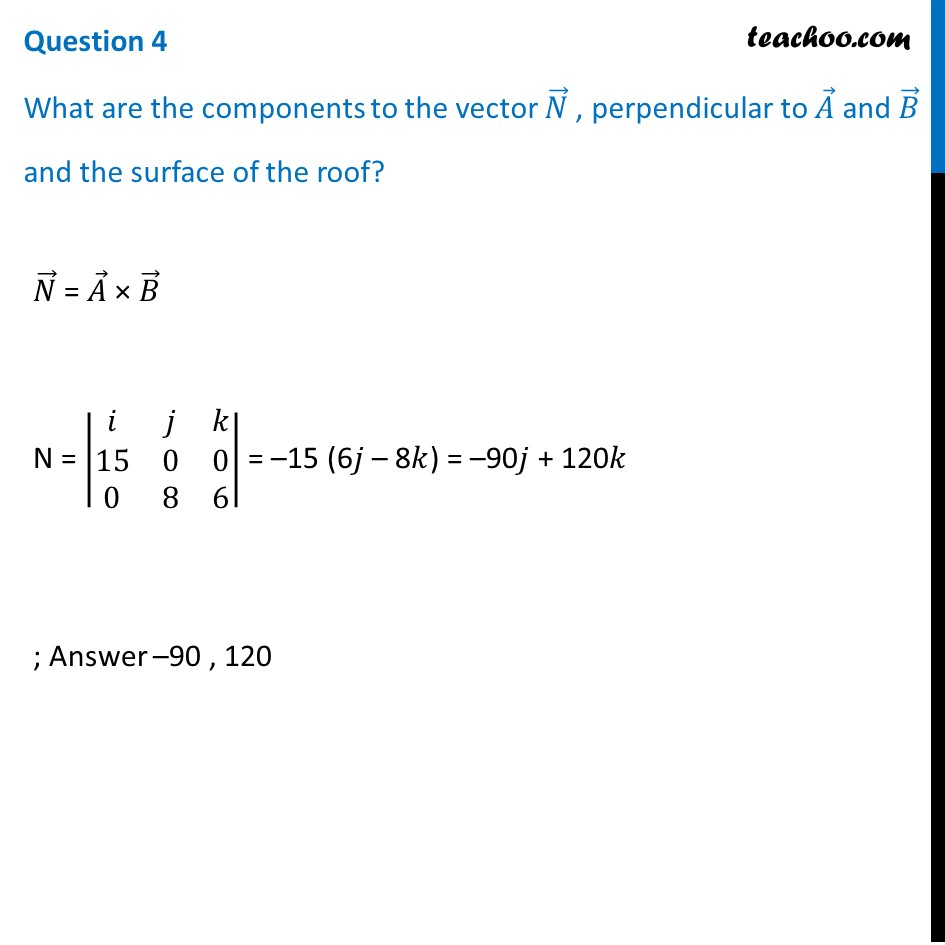## Question 5 What is the magnitude of N ⃗ and its units? The sun is located along the unit vector S ⃗  = 1/2 i ̂-6/7  j ̂+1/7  k ̂. If the flow of solar energy is given by the vector F ⃗  = 910 S in units of watts/meter2 , what is the dot product of vectors F ⃗ 𝑤𝑖𝑡ℎ N ⃗ , and the units for this quantity?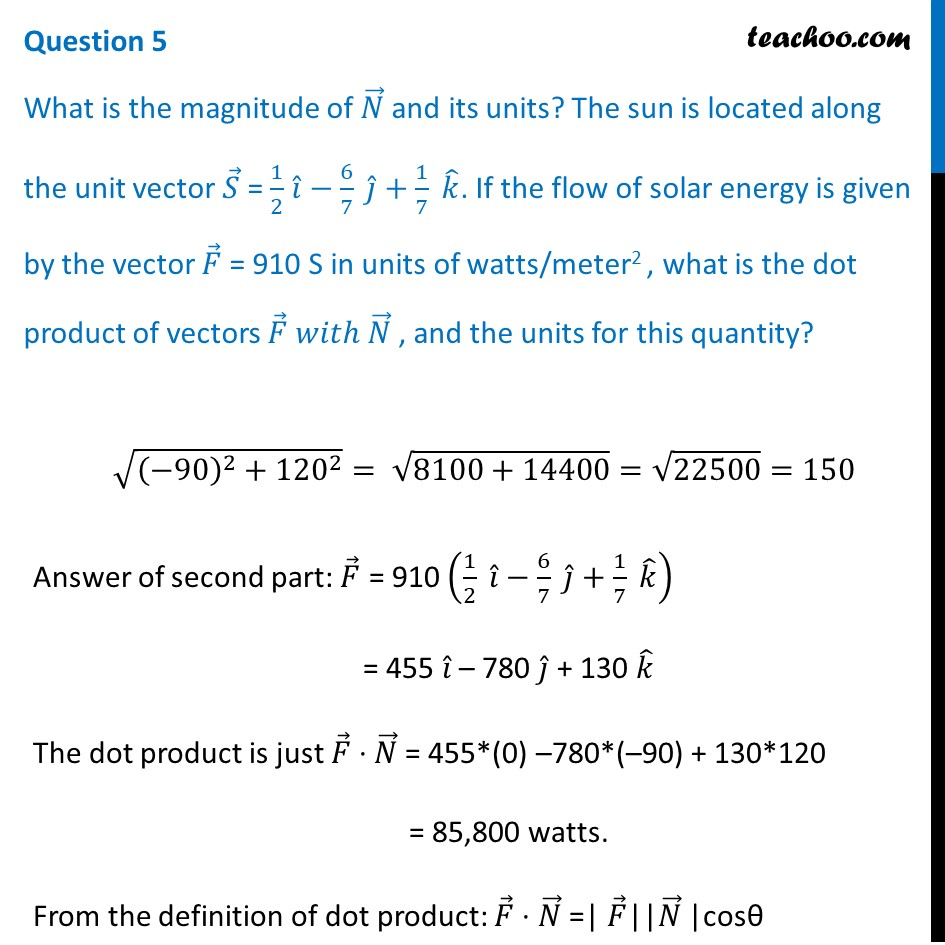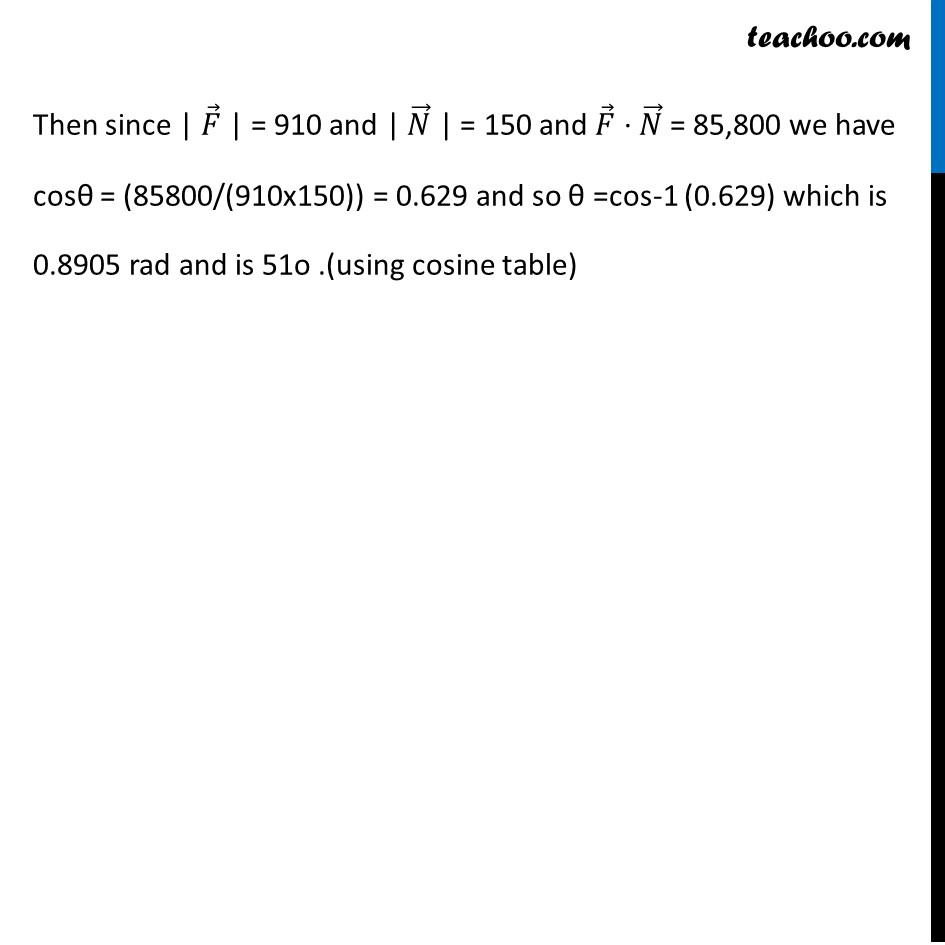## What is the angle between vectors N ⃗ and S ⃗ ? What is the elevation angle of the sun above the plane of the roof? (cos 51° = 0.629 )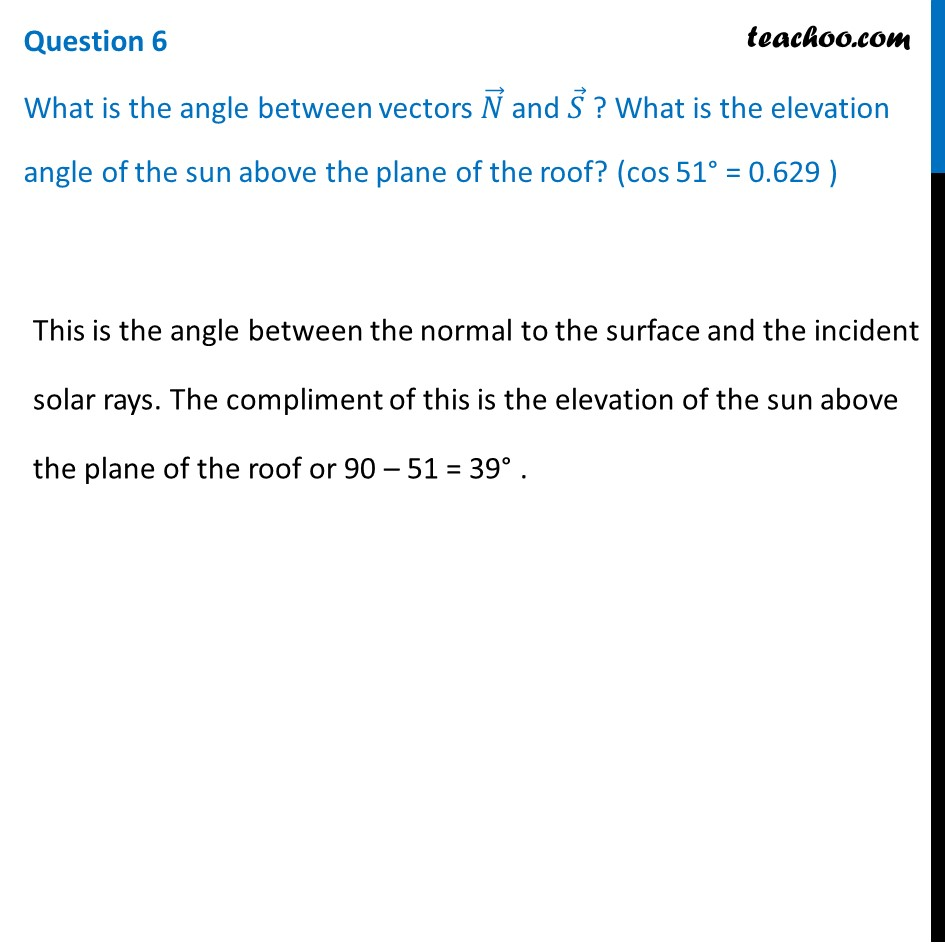Learn in your speed, with individual attention - Teachoo Maths 1-on-1 Class

### Transcript

Question Solar Panels have to be installed carefully so that the tilt of the roof, and the direction to the sun, produce the largest possible electrical power in the solar panels. A surveyor uses his instrument to determine the coordinates of the four corners of a roof where solar panels are to be mounted. In the picture , suppose the points are labelled counter clockwise from the roof corner nearest to the camera in units of meters P1 (6, 8, 4) , P2 (21, 8, 4), P3 (21, 16, 10) and P4 (6, 16, 10)Question 1 What are the components to the two edge vectors defined by 𝐴 ⃗ = PV of P2 – PV of P1 and 𝐵 ⃗ = PV of P4 – PV of P1? (where PV stands for position vector)15, 0, 0 : 0, 8, 6 Question 2 Write the vector in standard notation with 𝑖 ̂, 𝑗 ̂ and 𝑘 ̂ (where 𝑖 ̂, 𝑗 ̂ and 𝑘 ̂ are the unit vectors along the three axes).15i + 0j + 0k Answer 2: 0i + 8j + 6k Question 3 What are the magnitudes of the vectors 𝐴 ⃗ and 𝐵 ⃗ and in what units?Answer : 15 meters, Answer : √(8^2+6^2 ) = √(64+36) = √100 = 10 meters Question 4 What are the components to the vector 𝑁 ⃗ , perpendicular to 𝐴 ⃗ and 𝐵 ⃗ and the surface of the roof?𝑁 ⃗ = 𝐴 ⃗ × 𝐵 ⃗ N = |■8(𝑖&𝑗&𝑘@15&0&0@0&8&6)| = –15 (6𝑗 – 8𝑘) = –90𝑗 + 120𝑘 ; Answer –90 , 120 Question 5 What is the magnitude of 𝑁 ⃗ and its units? The sun is located along the unit vector 𝑆 ⃗ = 1/2 𝑖 ̂−6/7 𝑗 ̂+1/7 𝑘 ̂. If the flow of solar energy is given by the vector 𝐹 ⃗ = 910 S in units of watts/meter2 , what is the dot product of vectors 𝐹 ⃗ 𝑤𝑖𝑡ℎ 𝑁 ⃗ , and the units for this quantity?√((−90)^2+120^2 )= √(8100+14400)=√22500=150 Answer of second part: 𝐹 ⃗ = 910 (1/2 " " 𝑖 ̂−6/7 𝑗 ̂+1/7 𝑘 ̂ ) = 455 𝑖 ̂ – 780 𝑗 ̂ + 130 𝑘 ̂ The dot product is just 𝐹 ⃗ ∙ 𝑁 ⃗ = 455*(0) –780*(–90) + 130*120 = 85,800 watts. From the definition of dot product: 𝐹 ⃗ ∙ 𝑁 ⃗ =| 𝐹 ⃗||𝑁 ⃗ |cosθ Then since | 𝐹 ⃗ | = 910 and | 𝑁 ⃗ | = 150 and 𝐹 ⃗ ∙ 𝑁 ⃗ = 85,800 we have cosθ = (85800/(910x150)) = 0.629 and so θ =cos-1 (0.629) which is 0.8905 rad and is 51o .(using cosine table) Question 6 What is the angle between vectors 𝑁 ⃗ and 𝑆 ⃗ ? What is the elevation angle of the sun above the plane of the roof? (cos 51° = 0.629 ) This is the angle between the normal to the surface and the incident solar rays. The compliment of this is the elevation of the sun above the plane of the roof or 90 – 51 = 39° .